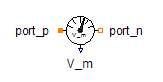Complex To Polar - MapleSim Help

Complex To Polar

Converts complex to polar representationDescription The Complex To Polar component converts the complex input to polar form.Equations $\mathrm{len}=\sqrt{{\mathrm{\Re }\left(u\right)}^{2}+{\mathrm{\Im }\left(u\right)}^{2}}$ $\mathrm{\phi }=\mathrm{arctan}\left(\mathrm{\Im }\left(u\right),\mathrm{\Re }\left(u\right)\right)$Connections

 Name Description Modelica ID $\mathrm{len}$ Real output; length of $u$ len $\mathrm{\phi }$ Real output; angle of $u$ phi $u$ Complex input uModelica Standard Library The component described in this topic is from the Modelica Standard Library. To view the original documentation, which includes author and copyright information, click here.